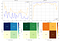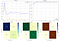# SMS Ham or Spam? SMS Classification NLP Modeling

Overview of Problem :

Short Message Service or SMS considered to be the text messaging service component of Telephone or Internet. In our day-to-day life we do receive considerable amount of SMS either from Friends, Telecom or Bank companies regarding our daily transactions or from tons of other sources. Some of these SMS texts are genuine whereas some can lead to fraudulent incidents.

Main task of this case study is making a Machine Learning model which can predict the SMS as HAM or SPAM with the help of text body of SMS. Dataset for this case study can be found at Kaggle. It consist of 5574 English text messages which are labeled as Ham or Spam.

Exploratory Data Analysis :

Main file provided in the problem is spam.csv which consist of SMS target label and Text Body.

We will be processing the text so as to remove any punctuation along with deconcatenation so as to make a complete word for model prediction.

Let is see the word cloud of Ham SMS text and Spam SMS text;

Word Cloud of Spam text can clearly show some unique words that appear in Text body, this mainly includes free, text, call, txt, mobile, reply etc.

Data Preparation :

First, we will load the data from provided csv file.

`df=pd.read_csv(’./raw_data/spam.csv’,       encoding="ISO-8859-1")[[’v1’, 'v2’]]`

## — Text Length of SMS Text :

Length of the complete text or words in the text can be considered to be the important feature for our analysis.

`def text_length(x):    return len(x.split())data['text_length'] = data['filtered_text'].map(text_length)data.head()`

We can clearly see Ham text with short text body or they have less text in it. On the other hand, Spam text tend to have lot of words, their mean text length is approximately 25 words.

## — Presence of Digit in Text body :

Presence of any numerical words can also considered to be much valuable feature for this classification. Spam texts tend to have numerical words as it can sometimes refer to a phone/mobile number or numbers in specific web link etc.

`def text_digit(x):    return len(re.findall(r'\d+', x))data['presence_of_digit'] = data['filtered_text'].map(text_digit)data.head()`

From above bar plots, we can see Ham text tend to have No numerical words or can have less number of words as count starts to increase. Distribution of this is more in case of Spam words as seen above.

By adding these two features along with processed text of SMS, we will make modeling for our SMS classification model.

Machine Learning Modeling

1. Text Vectorization :

As we already prepared our data, where we have, SMS text body, Text length and Presence of Digit in SMS Text as our features, we can make use of these features to predict the class label whether SMS is Spam or Ham (classification task).

For making the text understandable to the model, we need to convert the text into numerical form using Text Vectorization technique;

• Bag of Words Vectorization :
`#for uni-gram BoWbow        = CountVectorizer(min_df=5)bow.fit(x_tr['filtered_text'].values)bow_uni_tr = bow.transform(x_tr['filtered_text'].values)#for bi-gram BoWbow = CountVectorizer(min_df=5, ngram_range=(1, 2))bow.fit(x_tr['filtered_text'].values)bow_bi_tr = bow.transform(x_tr['filtered_text'].values)`
• TF-IDF Vectorization
`#for uni-gram TF-IDF vectorizationtfidf = TfidfVectorizer(min_df=5)tfidf.fit(x_tr['filtered_text'].values)tfidf_uni_tr = tfidf.transform(x_tr['filtered_text'].values#for Bi-gram TF-IDF vectorizationtfidf = TfidfVectorizer(min_df=5, ngram_range=(1, 2))tfidf.fit(x_tr['filtered_text'].values)tfidf_bi_tr = tfidf.transform(x_tr['filtered_text'].values)`
• Word Vectorer like Average Word2Vec and TF-IDF based Word2Vec

> Avg. Word2Vec can be made by taking avg of sum of word vectors of each words in the sentence.

`#creating corpus of words from given contexttext_corpus = list()for sent in tqdm(x_tr['filtered_text'].values):    text_corpus.append(sent.split())#text word2vectext_w2v = Word2Vec(text_corpus, min_count=5, size=w2v_dim, workers=4)#word2vec for sms textw2v_tr = text_to_w2v(x_tr)`

> TF-IDF Word2Vec on the other hand is, mean of sum of, TF-IDF value for each of the words in corpus multiplied by word vector value for that word.

`#train text corpustext_corpus = list()for sent in tqdm(x_tr['filtered_text'].values):    text_corpus.append(sent.split())#text word2vectext_tfidfw2v = Word2Vec(text_corpus, min_count=5, size=w2v_dim, workers=4)#processed review texttfidfw2v           = TfidfVectorizer(min_df=5)tfidfw2v_text      = tfidfw2v.fit_transform(x_tr['filtered_text'].values)tfidfw2v_text_dict = dict(list(zip(tfidfw2v.get_feature_names(), tfidfw2v.idf_)))#word2vec for sms texttfidfw2v_tr = text_to_tfidfw2v(x_tr)`

Combining these Word vector representation with remaining two features, we can finalize our data for modeling.

2. Evaluation Matrix :

We want our model to predict the spam messages very accurately and hence, we want True Positive to be high and False Positive very low. We can measure these two terms in the calculation of AUC (Area Under the Curve) and hence we will be using AUC as our metric for checking model performance.

As data is imbalances, we cannot simply use Accuracy as metric for model evaluation as any dumb model can high accuracy at the same time giving us very low True Positive Rate.

## — Naive Bayes Modeling :

Naive Bayes classifier is based on finding the probability of words with the help of Bayesian Probability of word given class label.

`nb_clf = MultinomialNB(alpha=alpha_value)nb_clf.fit(train, Y_train)pred = nb_clf.predict(cv)auc_score = roc_auc_score(Y_cv, pred)`

This model can be used with Uni-gram and Bi-gram BoW and TF-IDF vectorizers.

`from prettytable import PrettyTablenb = PrettyTable(['Model', 'Best Hyper-parameter', 'Train AUC', 'CV AUC', 'Test AUC'])for k, v in model_performances.items():    if k.startswith('Naive'):        nb.add_row([k, v, round(v, 5), round(v, 5), round(v, 5)])print(nb)`

## — Logistic Regression Model :

Assuming the data points of the dataset are linearly classified with hyper-plane, we can also model using Logistic Regression with Log Loss as loss function.

`lr_clf = SGDClassifier(loss='log', penalty='l2', alpha=alpha_value, random_state=27)lr_clf.fit(train, Y_train)pred = lr_clf.predict(cv)auc_score = roc_auc_score(Y_cv, pred)`

## — Tree Based Random Forest Model :

With the help of Tree based Random Forest classifier, we can also try to predict the performance of the model on given data.

`rf_clf = RandomForestClassifier(n_estimators=b_tree, max_depth=dep, criterion='gini', random_state=45, n_jobs=-1)rf_clf.fit(train, Y_train)pred = rf_clf.predict(cv)auc_score = roc_auc_score(Y_cv, pred)`

From all three models of different nature, we can clearly see that the Naive Bayes model performs better in all cases and with all vectorized cases with AUC value of more than 0.99.

Deep Learning Modeling

As we can see Machine Learning modeling works better with given data, we can also try Deep Learning Models like LSTM and simple Dense models to check the performance on data.

For text data, we can simply use Word Vector techniques as well as Word Embedding Layer to embed each word as Numerical representation and accordingly passing the same into deep learned layers.

1. Word Embedding :

We can tokenize each word of sentence with Tokenizer as below

`token = Tokenizer()token.fit_on_texts(x_tr['filtered_text'].values)#convert each eord in sentence to a word representationvocab_size   = len(token.word_index) + 1x_tr_encoded = token.texts_to_sequences(x_tr['filtered_text'].values)#pad each sentence with total of max (max_length) sized wordsx_tr_padded = pad_sequences(x_tr_encoded, maxlen=max_length, padding='post')`

## — Deep Learned Model :

Two Layered dense model was made to classify the vectorized SMS text to classify the same as Ham or Spam.

`model = Sequential()model.add(Dense(64, activation='relu', input_shape=(shape,)))model.add(Dropout(0.3))model.add(Dense(64, activation='relu'))model.add(Dropout(0.3))model.add(Dense(64, activation='relu'))model.add(Dropout(0.3))model.add(Dense(1))model.compile(loss='binary_crossentropy', optimizer=optimizers.Adam(lr=1e-3) ,metrics=[custom_auc])model_history = model.fit(x_tr_w2v, y_tr, batch_size=batch, epochs=epoch, validation_data=(x_cv_w2v, y_cv), use_multiprocessing=True, callbacks=[checkpoint_d1, stop_early_d1])`

Callbacks like Model Checkpoint, for storing best weights and Early Stopping, for terminating the training of model with best value was used to achieve best model for our task.Simple Dense Model : Train and CV performance & Test confusion Matrix

This model can be used with BoW and TF-IDF vectorizers of SMS text along with two Numerical features, i.e. Length of SMS Text and Presence of Number.

## — Simple LSTM Model :

LSTM, unlike Simple Deep Learning model, can hold the sequence information in text, hence can prove to be very good for classification task.

`model = Sequential()model.add(LSTM(64, activation='relu', input_shape=(shape, 1)))model.add(Dense(64, activation='relu'))model.add(Dropout(0.3))model.add(Dense(16, activation='relu'))model.add(Dropout(0.3))model.add(Dense(1))model.compile(loss='binary_crossentropy',optimizer=optimizers.Adam(lr=1e-3) ,metrics=[custom_auc])`

We will be using Avg. Word2Vec and TF-IDF Word2Vec based text encoded vectors.

`#getting data ready for LSTM modelx_tr_w2v_m = np.reshape(x_tr_w2v, (x_tr_w2v.shape, x_tr_w2v.shape, 1))model_history = model.fit(x_tr_w2v_m, y_tr, batch_size=batch, epochs=epoch, validation_data=(x_cv_w2v_m, y_cv), use_multiprocessing=True, callbacks=[checkpoint_d1, stop_early_d1])`

## — LSTM Model with Word Embedding :

Instead of converting text into vector with the help of Word Vector techniques, we can make use of Word Embedding layer in Tensorflow. With this approach, we can convert the text into series of Numerical numbers where each word will get unique number assigned to it. The weight of this embedding can either be trained or can be passed as predefined matrix.

`Embedding(vocab_size, 300, input_length=shape, trainable=True, name='text_embeding')lstm_layer1 = LSTM(64, name='lstm_layer1')(text_embeding)`

Output of Embedding layer can be passed to normal LSTM layer which can be further used to train Dense Layers. We trained Single and Two Layered LSTMs with the input from Embedding layer.LSTM with Text Embedding Layer : Train/CV Loss and AUC & Test Confusion Matrix

Conclusion :

Text vectorization plays the very important role in NLP tasks. Feature engineering from available text also plays important role in such classification.

Data provided with us is only 5574 text messages, which is small and imbalanced. Increasing the data size, can definitely make model robust as we get more words to train on.

Please refer complete code on GitHub.

ML/AI Enthusiast